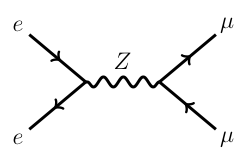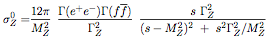# What are the line-shape parameters in resonance?

In particle physics, a resonance is the peak located around a certain energy found in cross sections of scattering experiments. These peaks are usually associated with subatomic particle (elementary or not) and their excitations. The peak energy of the resonance corresponds to the mass m of the particle. The width of the resonance (Γ), also called the particle decay width,  is related to the lifetime (τ) of the particle by the relation$\Gamma=\frac{\hbar}{\tau}$

where ħ is the reduced Planck constant. See also this answer for an intuitive explanation of this relation. For example, the Z boson has a mass of ~91.2 GeV and a width of ~2.5 GeV. When produced in e+e- collisions, through, e.g.,  the process depicted in the following graph,the number of Z (or better, the production cross section) varies with the centre-of-mass energy Ecm of the e+e- system as shown in the graph below (where the dots are values measured experimentally, and the line - or lineshape - is a prediction of the standard model):The resonance itself (with a centre-of-mass energy around the Z mass) is well visible and follows the Breit-Wigner formula:where s is the square of the centre-of-mass energy (i.e., 4 x E2beam). This formula contains all the measurable parameters of the resonance "lineshape". The lineshape itself is entirely contained in the third ratio, and depends solely on the mass and the width of the particle. The peak value of the cross section (12π Γee Γff / mZ2 ΓZ2 ) is obtained by setting s to MZ2 in the formula.

Measuring the lineshape of a resonance therefore correspond to measure the mass (i.e., the value of the centre-of-mass energy for which the cross section is maximum), the width of the Breit-Wigner around that mass (according to the above formula), and the peak cross section itself.

Category: